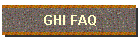####Question: How much material do I need for my project?

Answer: Take the total area, length times width, and divide it by 81 to get the cubic yards required for a four inch thick covering of that area. (Length X Width) / 81 = cubic yards (4 in. thick)

Question: How do I calculate the cubic yards I need for a six-inch thickness?

Answer: Take the answer to the above formula and multiply by 1.5. ((Length X Width) / 81) X 1.5 = cubic yards( 6 in. thick)

Question: How do I determine how many tons of crush and run I need?